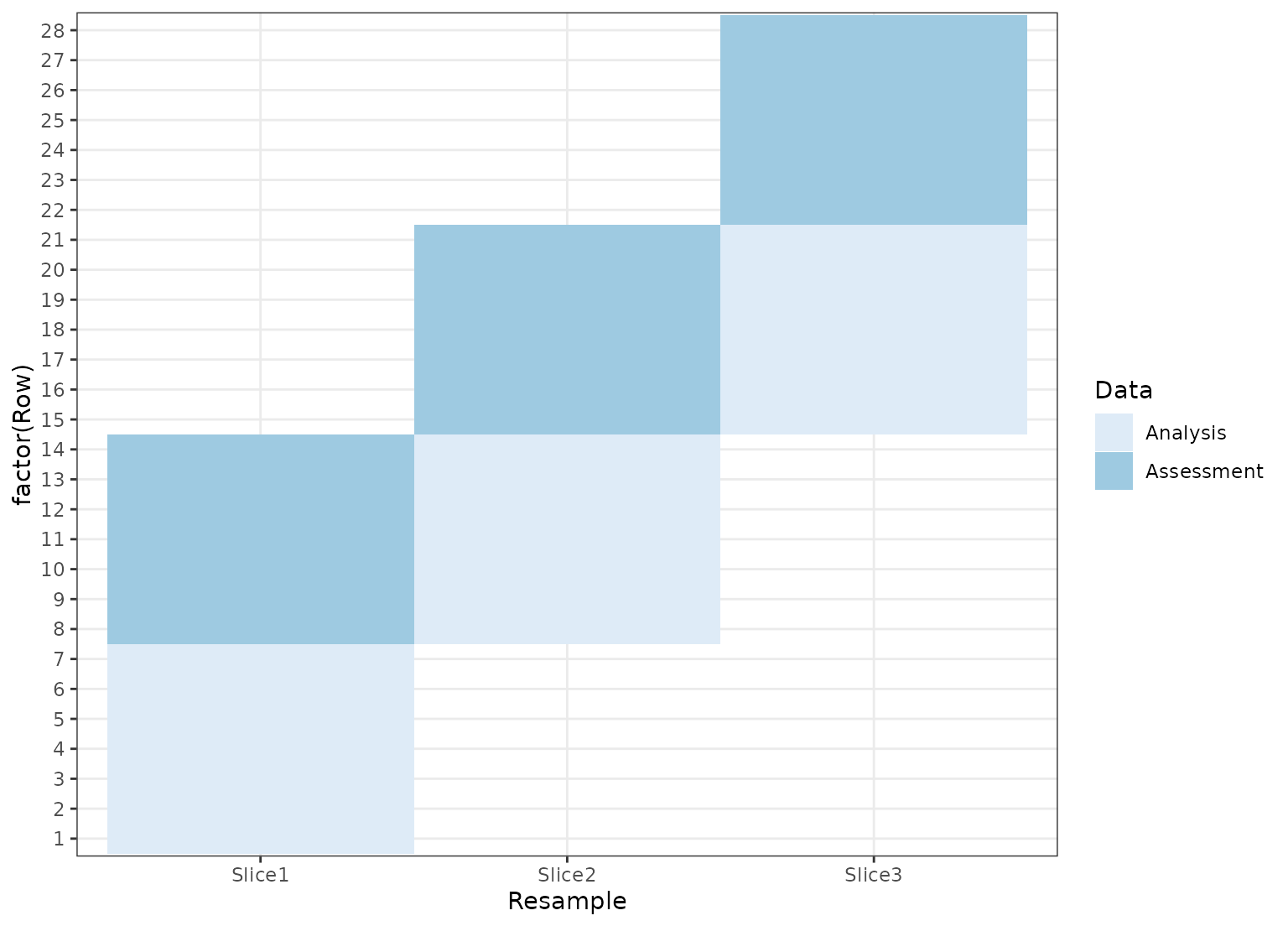The tidy function from the broom package can be used on rset and rsplit objects to generate tibbles with which rows are in the analysis and assessment sets.

## Usage

# S3 method for rsplit
tidy(x, unique_ind = TRUE, ...)

# S3 method for rset
tidy(x, ...)

# S3 method for vfold_cv
tidy(x, ...)

# S3 method for nested_cv
tidy(x, ...)

## Arguments

x

A rset or rsplit object

unique_ind

Should unique row identifiers be returned? For example, if FALSE then bootstrapping results will include multiple rows in the sample for the same row in the original data.

...

Not currently used.

## Value

A tibble with columns Row and Data. The latter has possible values "Analysis" or "Assessment". For rset inputs, identification columns are also returned but their names and values depend on the type of resampling. vfold_cv contains a column "Fold" and, if repeats are used, another called "Repeats". bootstraps and mc_cv use the column "Resample".

## Details

Note that for nested resampling, the rows of the inner resample, named inner_Row, are relative row indices and do not correspond to the rows in the original data set.

## Examples

library(ggplot2)
theme_set(theme_bw())

set.seed(4121)
cv <- tidy(vfold_cv(mtcars, v = 5))
ggplot(cv, aes(x = Fold, y = Row, fill = Data)) +
geom_tile() +
scale_fill_brewer()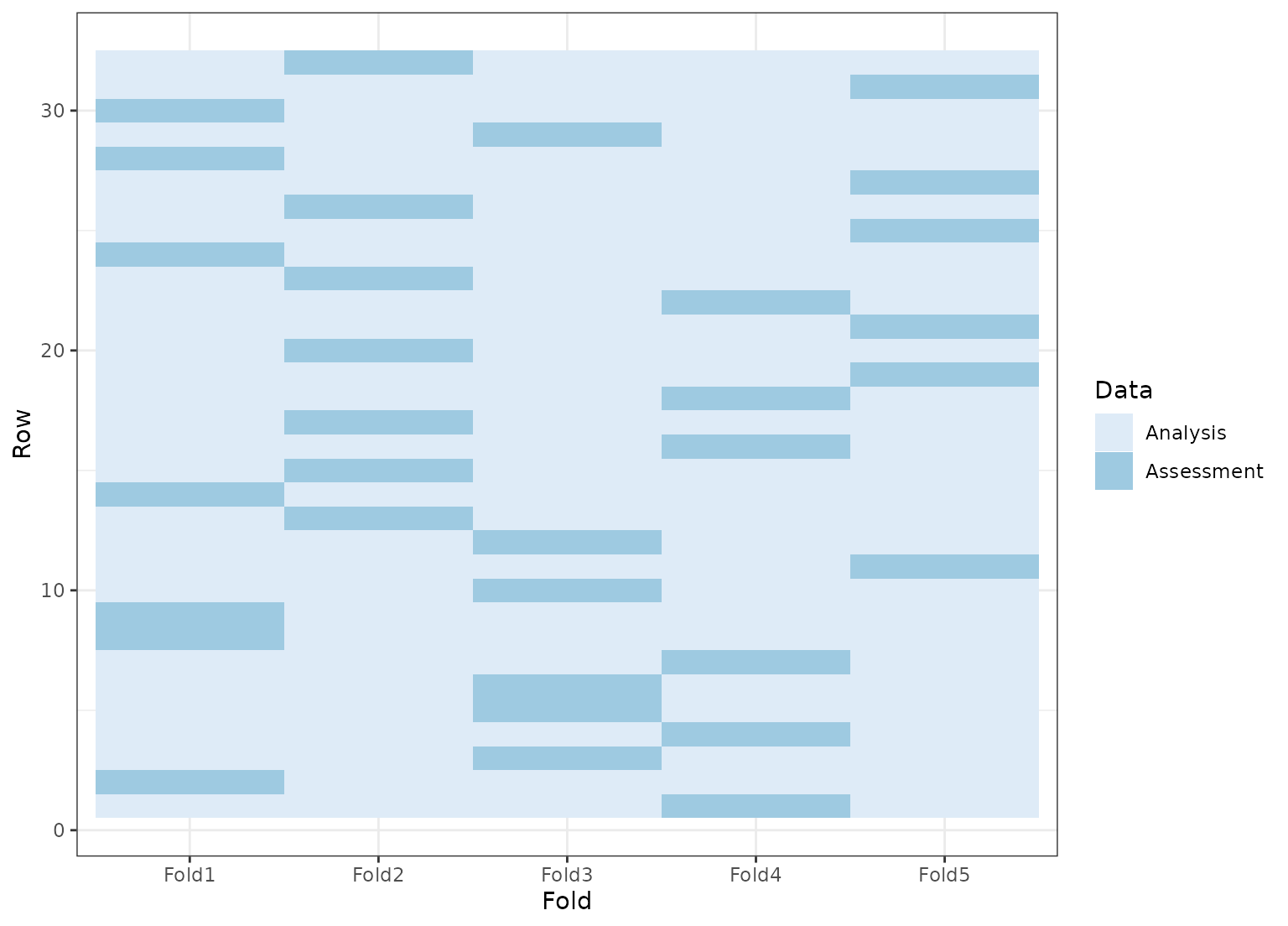set.seed(4121)
rcv <- tidy(vfold_cv(mtcars, v = 5, repeats = 2))
ggplot(rcv, aes(x = Fold, y = Row, fill = Data)) +
geom_tile() +
facet_wrap(~Repeat) +
scale_fill_brewer()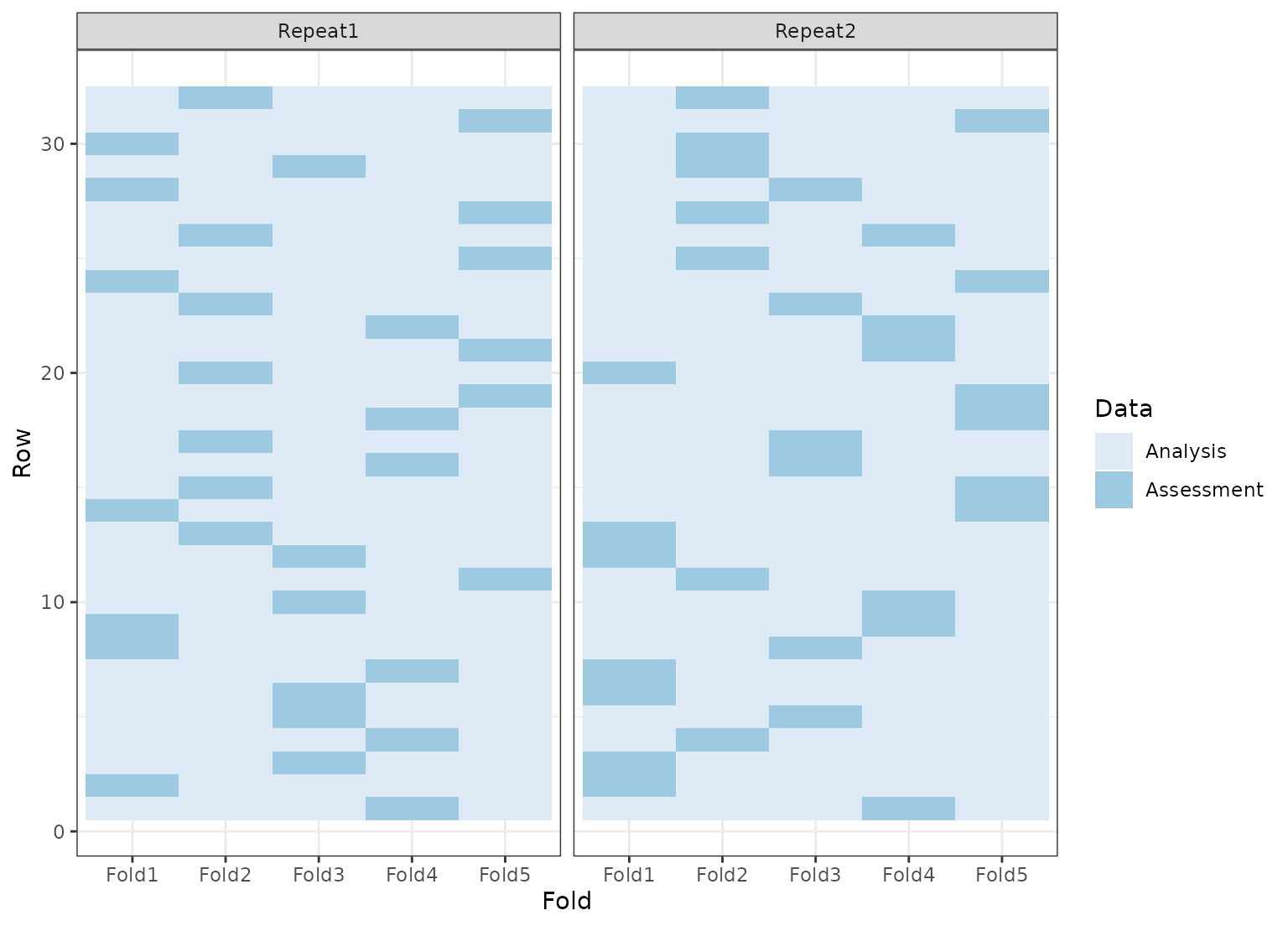set.seed(4121)
mccv <- tidy(mc_cv(mtcars, times = 5))
ggplot(mccv, aes(x = Resample, y = Row, fill = Data)) +
geom_tile() +
scale_fill_brewer()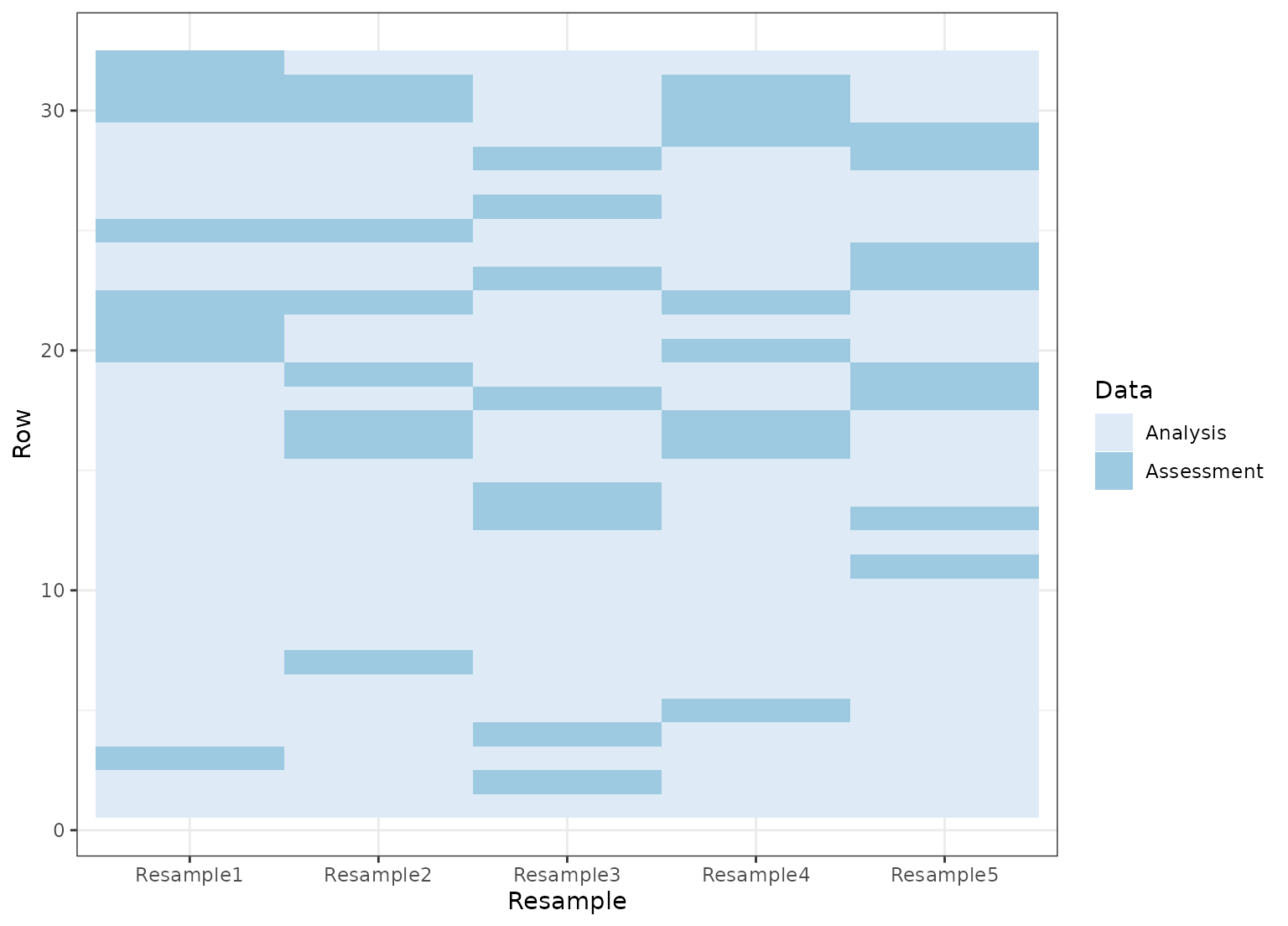set.seed(4121)
bt <- tidy(bootstraps(mtcars, time = 5))
ggplot(bt, aes(x = Resample, y = Row, fill = Data)) +
geom_tile() +
scale_fill_brewer()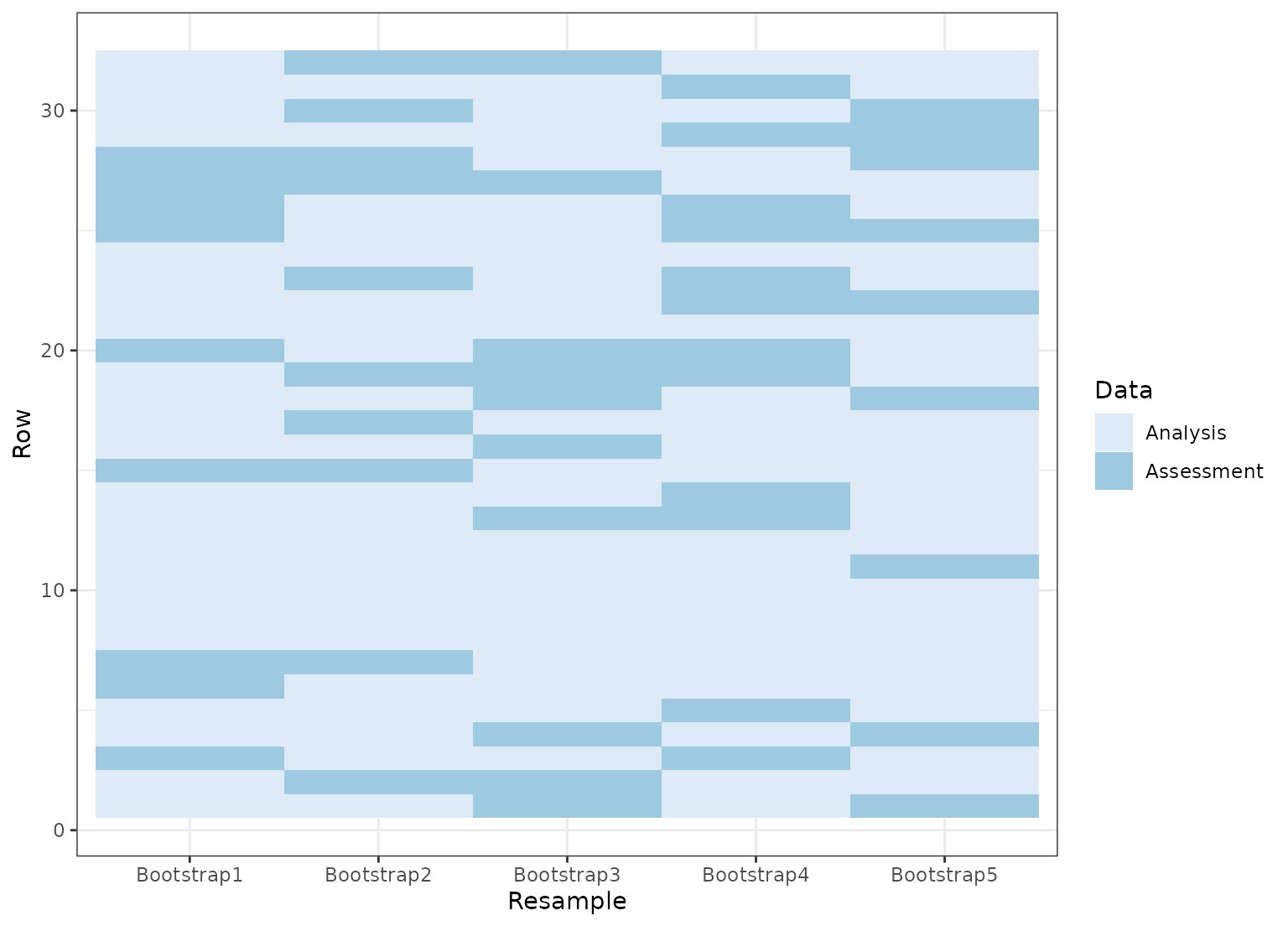dat <- data.frame(day = 1:30)
# Resample by week instead of day
ts_cv <- rolling_origin(dat,
initial = 7, assess = 7,
skip = 6, cumulative = FALSE
)
ts_cv <- tidy(ts_cv)
ggplot(ts_cv, aes(x = Resample, y = factor(Row), fill = Data)) +
geom_tile() +
scale_fill_brewer()